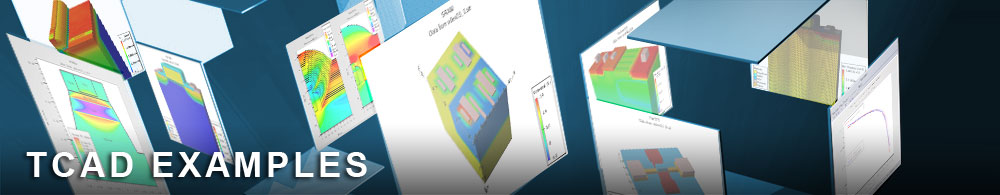Dynamic Response Simulation of Liquid Crystal

## clex24.in : Dynamic Response Simulation of Liquid Crystal

Requires: Victoryprocess/Clever/Tonyplot3D

Minimum Versions: VictoryProcess: 7.22.1.R/Clever 3.11.20.R
In this exampple, we will demonstrate how to simulate the dynamic LCD response using Clever LCD model. In dynamic LCD mode, LC directors's response to input signal is the key point in a given Liquid Crystal material and electrode configuration. To get the time response of LC material, we employ IPS(In-Plane-Switching) mode configuration. We demonstrate time dependent capacitance curve among electrodes and two dimensional transmittance contour at specific time where LC response is measured at rising, peak, and falling time. Addtionally, we simulated the transmittance curve with time which is very important characteristics of LCD.

Build 3D LCD structure using process simulator. First part of input is to make a 3D simulation structure using Victoryprocess process simulator. SpecifyMaskpoly statement define three pixel electrode and three common electrode which are normally grounded to zero. In Victoryprocess, Liquid crystal material is defined as user-defined material name "LC" and it is recognized as a liquid crystal material in Clever with liquid crystal parameters. Tonyplot3D plot show us generated three diemensional IPS(In-Plane-Switching) mode LCD structure. It consist of three Pixel electrodes and common electrodes.

Electrical & Optical simulation of LC is performed in Clever.
Before capacitance calculation, user need to define LC's anisotropic permittivity, elastic constant, and rotation viscosity.
setDynamicPixBias pixelfile="clex24_pulse.txt"

• it describes time pulse data from measurement file or other emulator.
Interconnect Capacitance domainboundarycondition=cyclic log="clex24_ips_3pix" structure="clex24_ips_3pix"
• performs field solver calculation and save to file at each time. Log="*" saves voltage of each electride as well as capacitance at simulated time.

As of post-processing, we plot pulsed pixel electrode voltage at each time, which are described in pixelfile="*" file. "Pix1" and "Pix2" are biased on 4V whiile "Pix2" is bias on zero voltage. As you can see in capacitance versus time plot, the time responsive capacitance curve of between "Pix2" and "Com" show us typical time delay as respect to input pulse.

To get optical properties of LC's response during time pulse, We assumed ideal linear polarizers with LC's refactive index at 550nm wavelength. "Optics" statement calculate the transmittance in given optical properties of material. We plot two dimensional transmittance contour at each time where "Pix2" electrode is biased on at corresponding pixel voltage at this time. To plot transmittance versus time, we extracted average transmittance value at each time and it is saved to clex24_0.dat file.

To load and run this example, select the Load example button in DeckBuild. This will copy the input file and any support files to your current working directory. Select the run button to execute the example.